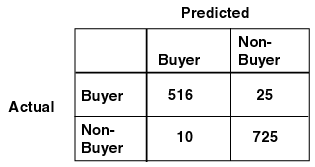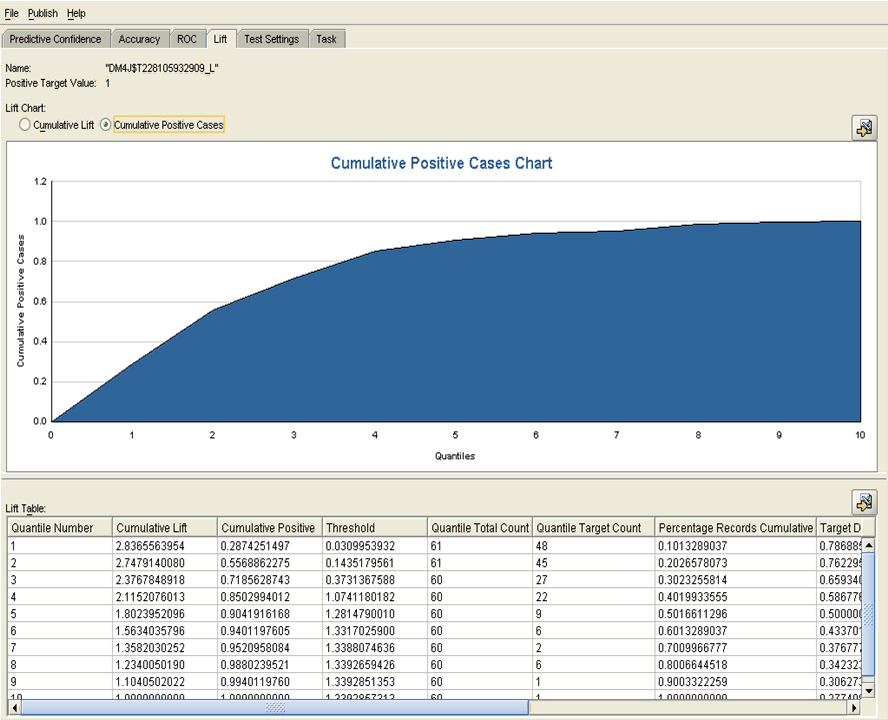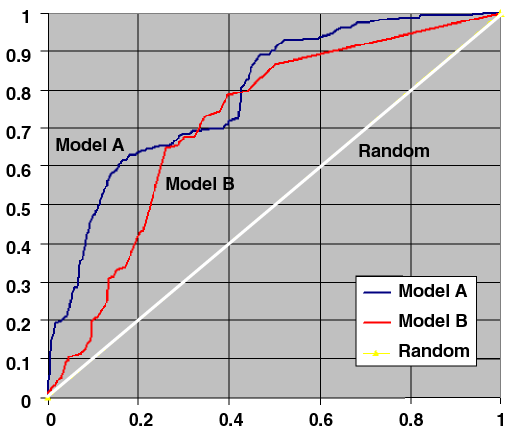# 5Classification

This chapter describes classification, the supervised mining function for predicting a categorical target.

"Supervised Data Mining"

This chapter includes the following topics:

Classification is a data mining function that assigns items in a collection to target categories or classes. The goal of classification is to accurately predict the target class for each case in the data.

A classification task begins with a data set in which the class assignments are known for each case. The classes are the values of the target. The classes are distinct and do not exist in an ordered relationship to each other. Ordered values would indicate a numerical, rather than a categorical, target. A predictive model with a numerical target uses a regression algorithm, not a classification algorithm.

For example, customers might be classified as either users or non-users of a loyalty card. The predictors would be the attributes of the customers: age, gender, address, products purchased, and so on. The target would be yes or no (whether or not the customer used a loyalty card).

In the model build (training) process, a classification algorithm finds relationships between the values of the predictors and the values of the target. Different classification algorithms use different techniques for finding relationships. These relationships are summarized in a model, which can then be applied to a different data set in which the class assignments are unknown.

Classification models are tested by comparing the predicted values to known target values. See "Testing a Classification Model".

### Binary and Multiclass Targets

The simplest type of classification problem is binary classification. In binary classification, the target attribute has only two possible values: for example, good credit risk or poor credit risk.

Multiclass targets have more than two values: for example, occupations such as engineer, teacher, or lawyer.

### Common Applications of Classification

Classification is used in customer segmentation, business modeling, credit analysis, and many other applications. For example, a credit card company might use a classification model to predict which customers are likely to pay their entire credit card balance every month. A medical researcher might use a classification model to predict patient response to a drug.

## Classification Algorithms

Oracle Data Mining provides the following algorithms for classification:

• Decision Tree

Decision trees automatically generate rules, which are conditional statements that reveal the logic used to build the tree. See Chapter 11, "Decision Tree".

• Naive Bayes

Naive Bayes uses Bayes' Theorem, a formula that calculates a probability by counting the frequency of values and combinations of values in the historical data. See Chapter 15, "Naive Bayes".

• Generalized Linear Models

Generalized Linear Models (GLM) is a popular statistical technique for linear modeling. Oracle Data Mining implements GLM in logistic regression for binary classification. See Chapter 12, "Generalized Linear Models".

• Support Vector Machines

Support Vector Machines (SVM) is a powerful, state-of-the-art algorithm based on linear and nonlinear regression. Oracle Data Mining implements SVM for binary and multiclass classification. See Chapter 18, "Support Vector Machines".

The nature of the data determines which classification algorithm will provide the best solution to a given problem. The algorithm can differ with respect to accuracy, time to completion, and transparency (See "Transparency"). In practice, it sometimes makes sense to develop several models for each algorithm, select the best model for each algorithm, and then choose the best of those for deployment.

## Biasing a Classification Model

Cost and benefit matrices and prior probabilities are methods for biasing a classification model.

Oracle Data Mining Application Developer's Guide to learn how to implement costs, benefits, and priors using the APIs

### Cost/Benefit Matrix

In a classification problem, it is often important to specify the cost or benefit associated with correct or incorrect classifications. Doing so can be valuable when the cost of different misclassifications varies significantly.

You can create a cost matrix to bias the model to minimize cost or maximize benefit. The cost/benefit matrix is taken into consideration when the model is scored.

For example, suppose the problem is to predict whether a customer will respond to a promotional mailing. The target has two categories: YES (the customer responds) and NO (the customer does not respond). Suppose a positive response to the promotion generates \$500 and that it costs \$5 to do the mailing. After building the model, you compare the model predictions with actual data held aside for testing. At this point, you can evaluate the relative cost of different misclassifications.

• If the model predicts YES and the actual value is YES, the cost of misclassification is \$0.

• If the model predicts YES and the actual value is NO, the cost of misclassification is \$5.

• If the model predicts NO and the actual value is YES, the cost of misclassification is \$495.

• If the model predicts NO and the actual value is NO, the cost is \$0.

Table 5-1 shows these relationships summarized in a cost matrix table.

Table 5-1 Cost Matrix

Actual Target Value Predicted Target Value Cost

YES

YES

`0`

NO

YES

`5`

YES

NO

`495`

NO

NO

`0`

The cost matrix shown in Table 5-1 shows that the cost of misclassifying a non-responder (sending the mailing to someone who does not respond) is only \$5.00, the cost of the mailing. However, the cost of misclassifying a responder (not sending the mailing to someone who would have responded) is \$495.00, because you will lose \$500.00 while only saving the cost of the mailing.

Using the same costs shown in Table 5-1, you can approach the relative value of outcomes from a benefits perspective. When you correctly predict a YES (a responder), the benefit is \$495. When you correctly predict a NO (a non-responder), the benefit is \$5.00 since you can avoid sending out the mailing. As the goal is to find the lowest cost solution, benefits would be represented as negative numbers in the matrix, as shown in Table 5-2.

Table 5-2 Cost/Benefit Matrix

Actual Target Value Predicted Target Value Cost/Benefit

YES

YES

`-495`

NO

YES

`0`

YES

NO

`0`

NO

NO

`-5`

"Cost-Sensitive Decision Making" in Oracle Data Mining Application Developer's Guide

### Priors

With Bayesian models, you can specify prior probabilities to offset differences in distribution between the build data and the real population (scoring data).

Note:

Priors are not used by the Decision Tree algorithm or logistic regression.

SVM classification uses priors as weights. See "Class Weights".

In many problems, one target value dominates in frequency. For example, the positive responses for a telephone marketing campaign may be 2% or less, and the occurrence of fraud in credit card transactions may be less than 1%. A classification model built on historic data of this type may not observe enough of the rare class to be able to distinguish the characteristics of the two classes; the result could be a model that when applied to new data predicts the frequent class for every case. While such a model may be highly accurate, it may not be very useful. This illustrates that it is not a good idea to rely solely on accuracy when judging a model.

To correct for unrealistic distributions in the training data, you can specify priors for the model build process. Table 5-3 shows a sample priors table that specifies a prior probability of 25% for a target value of 0 and 75% for a target of 1. This means that the ratio of 0 to 1 in the actual population is typically about 1 to 3.

Table 5-3 Priors Table

Target Value Prior Probability

`0`

25

`1`

`75`

Note:

The model should be tested against data that has the actual target values.

## Testing a Classification Model

A classification model is tested by applying it to test data with known target values and comparing the predicted values with the known values. The test data must be compatible with the data used to build the model and must be prepared in the same way that the build data was prepared.

### Confusion Matrix

A confusion matrix summarizes the types of errors that a classification model is likely to make. The confusion matrix is calculated by applying the model to test data in which the target values are already known. These target values are compared with the predicted target values.

A confusion matrix is a square with n dimensions, where n is the number of target classes. For example, a multiclass classification model with the target values `small`, `medium`, and `large` would have a three-by-three confusion matrix. A binary classification model has a two-by-two confusion matrix.

The rows of a confusion matrix identify the known target values. The columns indicate the predicted values.

Figure 5-1 shows a confusion matrix for a binary classification model. The target values are either `buyer` or `non-buyer`. In this example, the model correctly predicted a buyer 516 times and incorrectly predicted a buyer 10 times. The model correctly predicted a non-buyer 725 times and incorrectly predicted a non-buyer 25 times.

Figure 5-1 Sample Confusion MatrixDescription of "Figure 5-1 Sample Confusion Matrix"

The following can be computed from this confusion matrix:

• The model made 1241 correct predictions (516 + 725) .

• The model made 35 incorrect predictions (25 + 10).

• The model scored 1276 cases (1241+35).

• The error rate is 35/1276 = 0.0274.

• The accuracy rate is 1241/1276 = 0.9725.

### Lift

Lift measures the concentration of positive predictions within segments of the population and specifies the improvement over the rate of positive predictions in the population as a whole.

Lift is commonly used to measure the performance of targeting models in marketing applications. The purpose of a targeting model is to identify segments of the population with potentially high concentrations of positive responders to a marketing campaign. Lift is the ratio of positive responders in a segment to the positive responders in the population as a whole. For example, if a population has a predicted response rate of 20%, but one segment of the population has a predicted response rate of 60%, then the lift of that segment is 3 (60%/20%).

The notion of lift implies a binary target: either a responder or not a responder, either yes or no. Lift can be computed for multiclass targets by designating a preferred positive class and combining all other target class values, effectively turning a multiclass target into a binary target.

The calculation of lift begins by applying the model to test data in which the target values are already known. Then the predicted results are sorted in order of probability, from highest to lowest predictive confidence. The ranked list is divided into quantiles (equal parts). The default number of quantiles is 10.

Oracle Data Mining computes the following lift statistics:

• Probability threshold for a quantile n is the minimum probability for the positive target to be included in this quantile or any preceding quantiles (quantiles n-1, n-2,..., 1). If a cost matrix is used, a cost threshold is reported instead. The cost threshold is the maximum cost for the positive target to be included in this quantile or any of the preceding quantiles.

• Cumulative gain for a quantile is the ratio of the cumulative number of positive targets to the total number of positive targets.

• Target density of a quantile is the number of true positive instances in that quantile divided by the total number of instances in the quantile.

• Cumulative target density for quantile n is the target density computed over the first n quantiles.

• Quantile lift is the ratio of target density for the quantile to the target density over all the test data.

• Cumulative percentage of records for a quantile is the percentage of all test cases represented by the first n quantiles, starting at the end that is most confidently positive, up to and including the given quantile.

• Cumulative number of targets for quantile n is the number of true positive instances in the first n quantiles.

• Cumulative number of nontargets is the number of actually negative instances in the first n quantiles.

• Cumulative lift for a quantile is the ratio of the cumulative target density to the target density over all the test data.

The sample lift chart in Figure 5-2 shows that the cumulative lift for the top 30% is 2.37. The next column indicates that over 71% of all likely positive responses are found in the top 3 quantiles.

Figure 5-2 Sample Lift ChartDescription of "Figure 5-2 Sample Lift Chart"

ROC is a method for experimenting with changes in the probability threshold and observing the resulting effect on the predictive power of the model.

ROC curves are similar to lift charts in that they provide a means of comparison between individual models and determine thresholds which yield a high proportion of positive hits. ROC was originally used in signal detection theory to gauge the true hit versus false alarm ratio when sending signals over a noisy channel.

The horizontal axis of an ROC graph measures the false positive rate as a percentage. The vertical axis shows the true positive rate. The top left hand corner is the optimal location in an ROC curve, indicating high TP (true-positive) rate versus low FP (false-positive) rate.

The area under the ROC curve (AUC) measures the discriminating ability of a binary classification model. The larger the AUC, the higher the likelihood that an actual positive case will be assigned a higher probability of being positive than an actual negative case. The AUC measure is especially useful for data sets with unbalanced target distribution (one target class dominates the other).

In the example graph in Figure 5-3, Model A clearly has a higher AUC for the entire data set. However, if a false positive rate of 40% is acceptable, Model B is better suited, since it achieves a better error true positive rate at that false positive rate.

Figure 5-3 Receiver Operating Characteristics CurvesDescription of "Figure 5-3 Receiver Operating Characteristics Curves "

Besides model selection the ROC also helps to determine a threshold value to achieve an acceptable trade-off between hit (true positives) rate and false alarm (false positives) rate. By selecting a point on the curve for a given model a given trade-off is achieved. This threshold can then be used as a post-processing parameter for achieving the desired performance with respect to the error rates. Data Mining models by default use a threshold of 0.5.

Oracle Data Mining computes the following ROC statistics:

• Probability threshold: The minimum predicted positive class probability resulting in a positive class prediction. Different threshold values result in different hit rates (`true_positive_fraction`) and different false alarm rates (`false_positive_fraction`).

• True negatives: Negative cases in the test data with predicted probabilities strictly less than the probability threshold (correctly predicted).

• True positives: Positive cases in the test data with predicted probabilities greater than or equal to the probability threshold (correctly predicted).

• False negatives: Positive cases in the test data with predicted probabilities strictly less than the probability threshold (incorrectly predicted).

• False positives: Negative cases in the test data with predicted probabilities greater than or equal to the probability threshold (incorrectly predicted).

• True positive fraction: Hit rate. (true positives/(true positives + false negatives))

• False positive fraction: False alarm rate. (false positives/(false positives + true negatives))